Announcements

# PSoC™ 4 Forum Discussions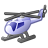Level 1
Level 1# How to convert raw count value in capacitance [pF] ?

Hi everybody,

I'm using the development board CY8CKIT-040 with the chip CY8C4014LQI-422.

I would like to convert the raw count value into a capacitance value in pF. Looking to the document No. 001-64846 Rev. *X I have found Equation 6 that relates Raw Counts with Cs but it is not totally clear to me.

I don't understand what is the unit of measure of Cs and if Fsw is referring to the maximum sense clock frequency or to the average. I'm using the manual tuning so all the parameters are fixed by me (Icomp, Imod, ...).

Thanks,

Fede

18 RepliesModeratorModerator# Re: How to convert raw count value in capacitance [pF] ?

Hi Fede,

If you want to get the sensor Cp, you can use component Built in self test function.

Cs represent the sum of  parasitic capacitance and finger capacitace.

The unit of the Cs is f.

Fsw equals to the HFCLK/sensor clock divider.

If you want to know more about the CapSense , you can refer to below linker file CapSense Desing guide:

The doc clarifies how to set the system parameter.

Hope it can be helpful for you.

Thanks,

LinglingLevel 1
Level 1# Re: How to convert raw count value in capacitance [pF] ?

Hi Lingling,

I have checked all the parameters in my Cypress but there is something that is still not clear.

I'm using the Capsense v6, I have a PCB with 4 copper buttons connected to the Cypress and I'm using these parameters:

Imod 29; Icomp 25; Vref 1.2 V; Fsw (HFClk) 12 MHz; n 16 bit.

During the measurements I have a Cp of 105 pF (measured with CapSense_GetSensorCapacitance), without a finger I'm expecting to have more or less the same result from the formula but the calculation with these parameters results Cs = 2.97E-06. What I'm doing wrong?

Thank youLevel 1
Level 1# Re: How to convert raw count value in capacitance [pF] ?

Any other answer to my reply? Do you know why the formula is not working?ModeratorModerator# Re: How to convert raw count value in capacitance [pF] ?

I doubt that the value of Cp 105 is wrong.

Do you enable any filters in your project?

In sourcing mode:

raw count = 2N * VREF* FSW*CS/ IMOD  − 2N * ICOMP/ IMOD

In sinking mode:

raw count = 2N * (VDD −  VREF)* FSW *  CS /IMOD− 2N * ICOMP/ IMOD

Imod=29*1.2uA

Icomp=25*1.2uALevel 1
Level 1# Re: How to convert raw count value in capacitance [pF] ?

I think that 105 pF is the right capacity, it is what I expect from the sensor design and it is similar to a measurement taken using an impedance

analyzer.

In the formula, I didn't multiply Imod and Icomp by 1.2 but in any case, the result that I obtain is no sense: 3.56E-06 is too high!

I'm using the IIR filter of the 1st order and I have the self-test library activated to measure the Cp. I have the IDAC sourcing mode.

The data that I'm using in the formula are the same as before:

Imod = 29

Icomp = 25

Fsw =  12000000

Vref = 1.2

n = 16

Rawcount = 40000

The result is wrong with some order of difference (E-04), I think that some number that I put in the formula is wrong... perhaps Fsw?

ThanksLevel 3
Level 3# Re: How to convert raw count value in capacitance [pF] ?

Hi,

I have a problem similar to yours.
I think that Fsw is not equal to 12 000 kHz but lower because Fsw it is the frequency of the sense clock, not the modulator clock.
(Could be 6000-3000-1500-750-375.... kHz)

AnmaLevel 1
Level 1# Re: How to convert raw count value in capacitance [pF] ?

Thank you for the reply. I think the same, Fsw should be the Sense Clock Frequency as written in the document No. 001-64846 Rev. *X but the formula is still not working. Starting from the Rawcount formula with Idac in sourcing mode:

Cs = (Imod*Rawcount)/((2N-1)*Vref*Fsw) + Icomp/(Vref*Fsw)

So with my values (using Fsw = 750 KHz instead of the Modulator clock frequency) I obtain Cs = 5,69E-05 F that is really far from 105 pF (the parassitic capacitance read by Cypress, Cf is 0 because there isn't any finger over the sensor).

I'm quite sure about Imod, Icomp, N because are set by me with the tuner and I can read that values during the measurements... Vref is fixed at 1.2 so I think that Fsw is something different to have the formula working... But I don't understand what could be the right value. Or is the formula that is wrong.

I hope that someone from Cypress' team can help us soon.

FedeModeratorModerator# Re: How to convert raw count value in capacitance [pF] ?

Hi anma_3197476​,

1. PSoC 4000 device has 3rd gen CapSense in it. The supported parasitic capacitance is only from 5 - 60 pF. 105 pF is too high value of Cp for this device. Could you please let us know the dimensions of the button, trace width and trace length.

2. Can you please check the if you are using auto mode/ PRS as sense clock source? If yes, you need to use the avg sense clock frequency as displayed by the component which is Fsw/ 2. To avoid confusion you can use direct method and use the same Fsw. Also while measuring Cp make sure you have set sense clock as direct.

3. Could you please provide the raw counts that you obtained so that we can back calculate it from our side and compare our results.

Regards,

Regards,Level 3
Level 3# Re: How to convert raw count value in capacitance [pF] ?

Well, I have set up the following configuration:

• CYCKIT040 using the following hardware config:

22.3 pf capacitor connected between pin p0 and pcb ground as "Verification capacitor"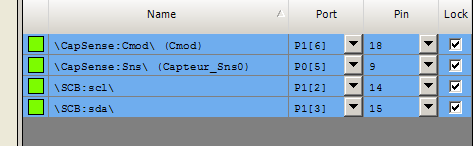• Capsense ver 5.0 with the following software config: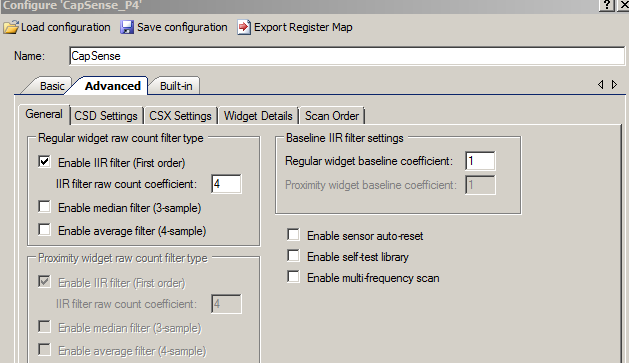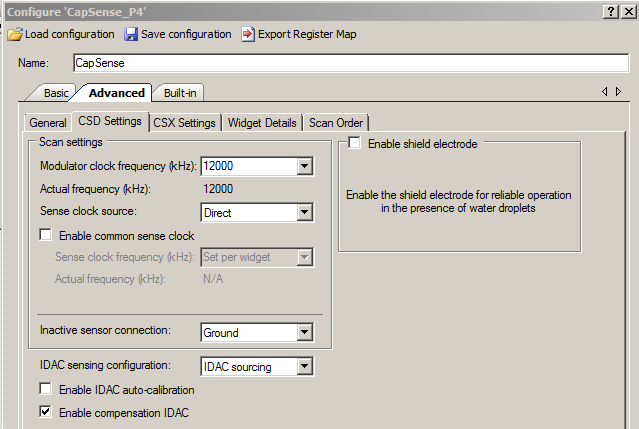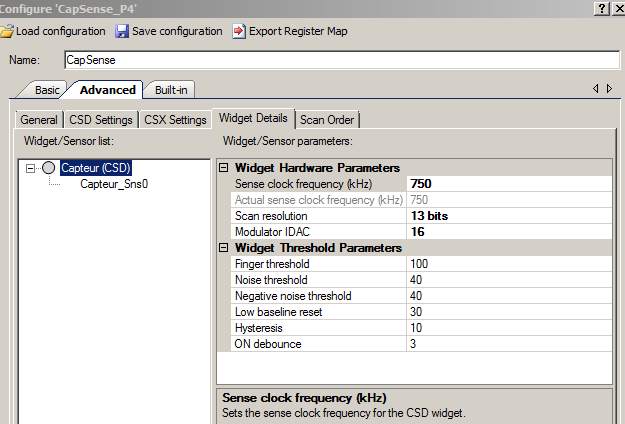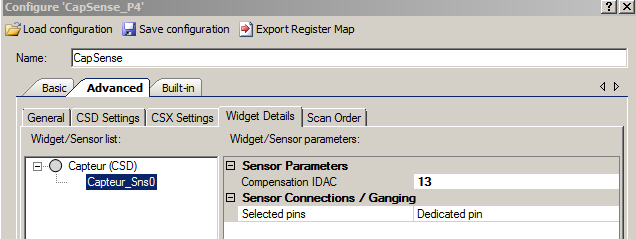• Formula used, according to CYPRESS documentation  0001-85931

Raw Counts =(Max_Counts*Vref*Fsw*1000*Cs)/(Imod*1000000000000)-(Max_Counts*Icomp/Imod)
Purpose is to check if the Raw counts correctly processed gives the right value of the known capacitor.

Where:
Max_Counts=8192

Vref= 1.2
Fsw=750

Cs= 22.3
1000 is a conversion factor from (kHz <> Hz)
1000000000000 is another conversion factor (pf <> F)

Imod=  0.0000192  (Amps here)
Icomp=0.0000156  (Amps here)

• Raw counts read is 7115 counts and the above formula gives 1907 counts: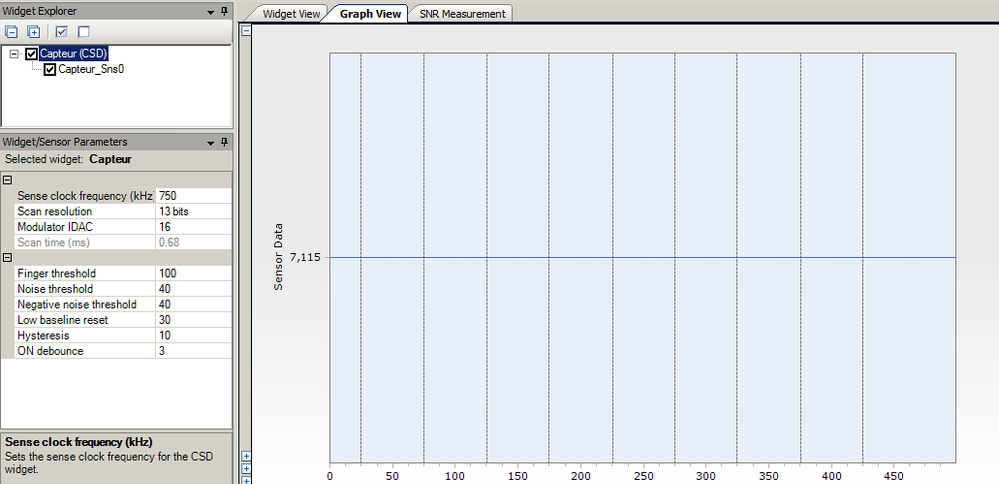So, where did I made a mistake ?

Thanks to the one that will bring me the light of knowledge !
AnmaModeratorModerator# Re: How to convert raw count value in capacitance [pF] ?

One observation from your screenshot. CMOD is connected to 0.4 in the kit CY8CKIT-040. You have used 1.6 pin Can you please check that once?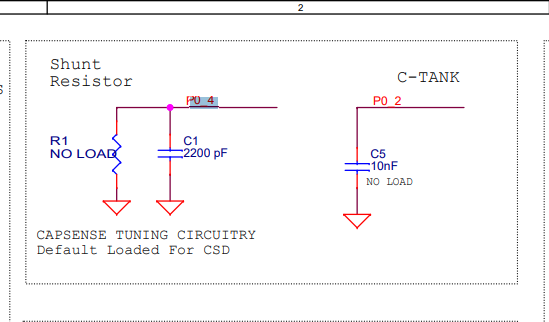Can you please turn on IDAC auto calibration and then check your IDAC values as reported in the tuner. Use these values for comp IDAC and mod IDAC. But the auto calibration routine will set the mod IDAC and comp IDAC to 85 %. I can see that you have set it to around 23 %. It is recommended to set calibration percent to 85 %. Also there are issues with mod IDAC values less than 20. Please set mod IDAC values greater than 20 and then set the comp IDAC to get 85 % of  max raw counts.

Regards,

Regards,Level 3
Level 3# Re: How to convert raw count value in capacitance [pF] ?

CMOD is now connected on P0. Then I set IDAC autocalibration to ON, compile, flash and launch tuner.

I got Imod=16 and Icomp=14 and Raw counts= 0 !
It seems that the calibration routine has given me values lower then 20.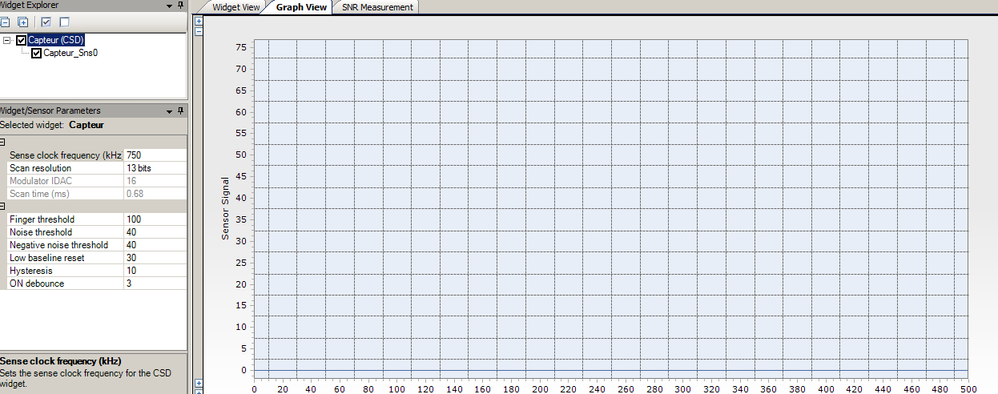Then, I just turned IDAC autocalibration to OFF, keeping Imod=16 and Icomp=14. After compile and flash, I got Raw counts=55 !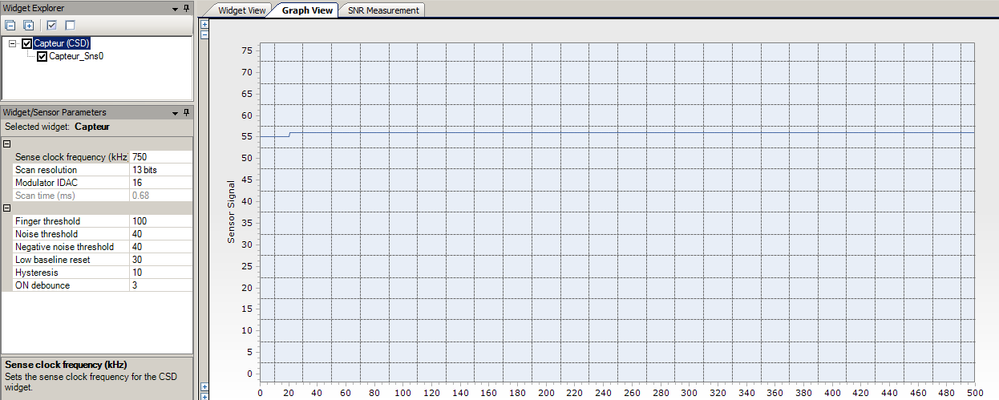Still stuck for the present time.Level 3
Level 3# Re: How to convert raw count value in capacitance [pF] ?

Now, will make another test following this:

 Please set mod IDAC values greater than 20 and then set the comp IDAC to get 85 % of  max raw counts.Level 3
Level 3# Re: How to convert raw count value in capacitance [pF] ?

So, 22.3 pF capacitor still in place with following setting: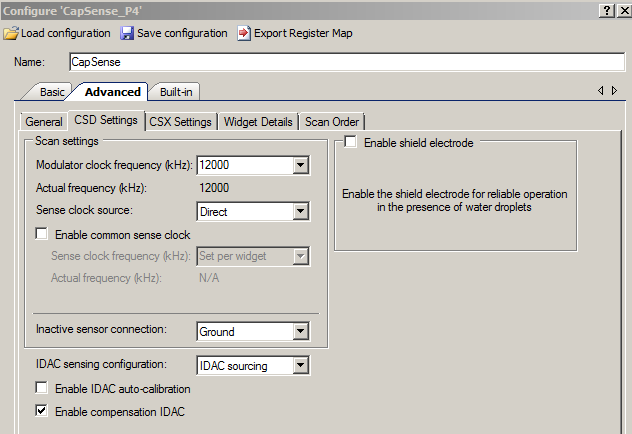then, Imod is set to 21 and Icomp is increased until getting around 6963 counts on the graph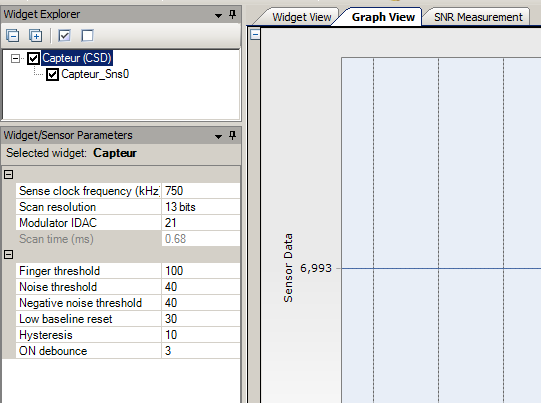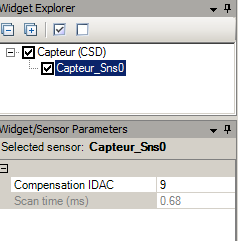In this case, when Icomp=9 we have a raw count of 6993, which is close to 6963. (85% limit)

Formula 3-8 from manual 0001-85931 gives 3013 counts, which is not correct !!

Any idea ?Level 3
Level 3# Re: How to convert raw count value in capacitance [pF] ?

Any expert from Cypress here ?ModeratorModerator# Re: How to convert raw count value in capacitance [pF] ?

Can you to check the results using SmartSense? SmartSense will first calculate the Cp of the sensor and will set all the hardware and software parameters accordingly. Can you check this let us know if it works.

Also, probe the CMOD pin and check if you o​bserve Vref in it. Probe you sensor and check if you can see proper charging and discharging waveform?

Regards,

Regards,Level 3
Level 3# Re: How to convert raw count value in capacitance [pF] ?

 Can you to check the results using SmartSense? SmartSense will first calculate the Cp of the sensor and will set all the hardware and software parameters accordingly. Can you check this let us know if it works.
• Now with SMARTSENSE activated and sense clock= Direct, looking in tuner gives the following values:
Fsw= 1500 kHz
Resol= 15 bits
Modulator= 63
Raw counts= 27930

• Now with MANUAL activated, IDAC auto-calibration and compensation both disabled, looking in tuner gives the following values:

Fsw= 1500 kHz

Resol= 15 bits

Modulator= 63

Raw counts= 27934

• Raw counts checking using formula: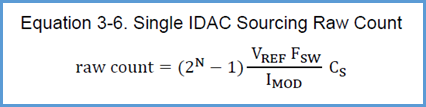Using the formula above, I am getting 17398 counts instead of about 27934. !!!

 Also, probe the CMOD pin and check if you observe Vref in it. Probe you sensor and check if you can see proper charging and discharging waveform?

That's the plan for tomorrow...

AnmaLevel 3
Level 3# Re: How to convert raw count value in capacitance [pF] ?

I kept the same parameters that previous message and made some measurements:

Here is the signal measured on CMOD (pin 0.4). Showing 1.2 volts DC stable: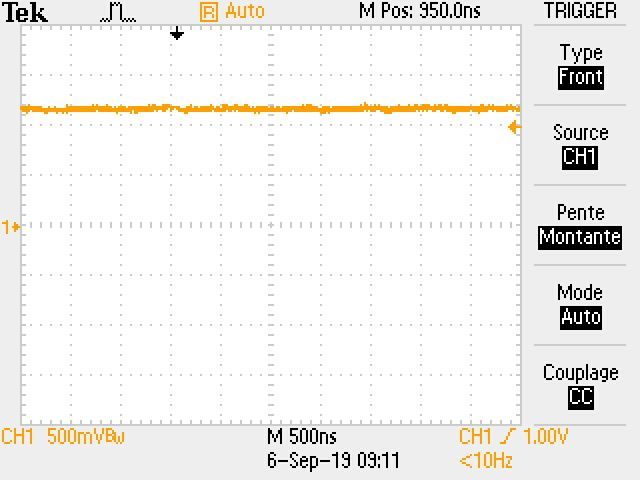Here is the signal measured on Csense (pin 0.5). Showing 1.2 volts a 1500 kHz frequency.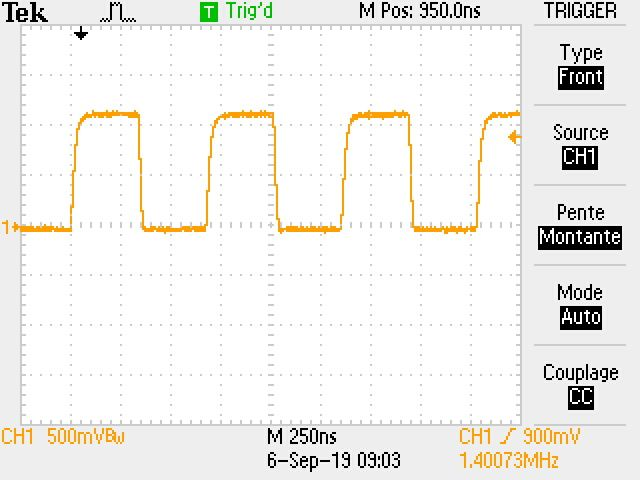During this time, I had a look on the raw counts curve and the input capacitance of the scope changes the things, buts that's normal IMHO.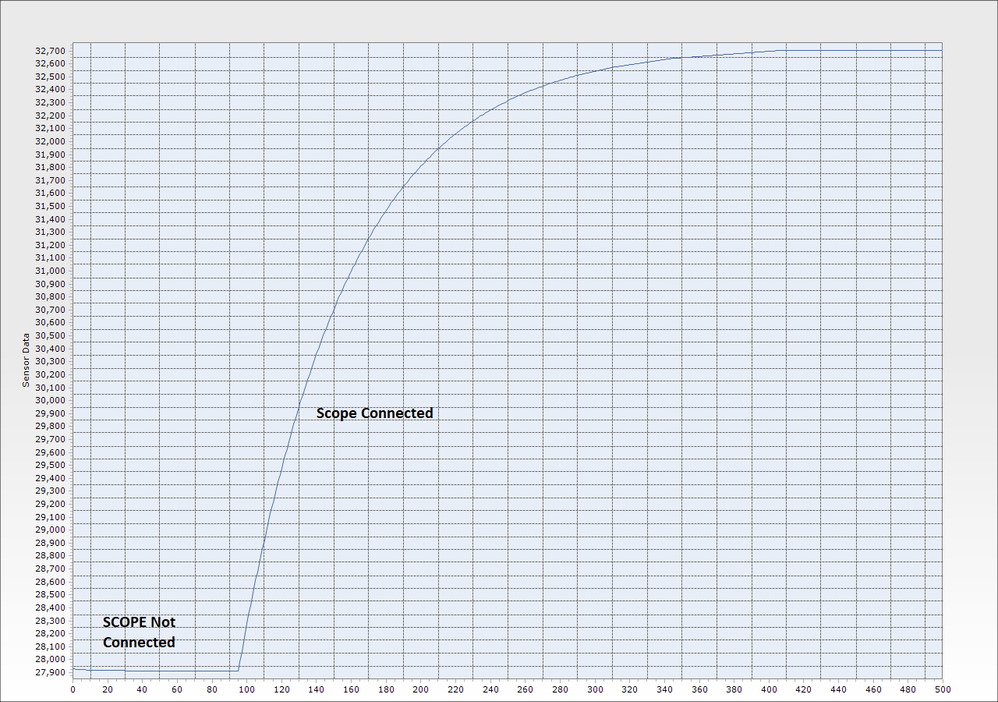So, this doesn't explain why the formula doesn't give the right raw counts value for a know capacitor !!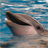Employee
Employee

# Re: How to convert raw count value in capacitance [pF] ?

Hi Anma,

Please, check your calculations. If we calculate Cs from the rawcounts formula for SmartSense parameters, we obtain Cs = Rawcounts x Imod / ((2^N - 1)  x Fsw x Vref) = 27930 x 63 x 1 200 000 pA / ((2^15 - 1) x 1 500 kHz x 1200 mV) = 35,8 pF. This value is more similar to real values than 105 pF. The more common sensor capacity is 5 - 25 pF depending on a configuraion and a trace.

Best regards,

Andriy